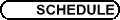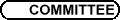SS17 - Systèmes dynamiques complexes / SS17 - Complex Dynamical Systems
Org: X. Buff (Toulouse), A. Cheritat (Toulouse) et/and M. Yampolsky (Toronto)

ARTUR AVILA, College de France

ILIA BINDER, University of Toronto
Multifractal Analysis of Harmonic Measure

We discuss the multifractal analysis of a planar harmonic measure and the boundary rotation. We are especially interested in the sharp bounds for the local dimension of harmonic measure and the rate of rotation, the so-called universal spectrum. The theory is well studied for simply connected domains, with the help of the conformal mapping techniques. It is also well established for hyperbolic polynomial Julia sets, by the means of thermodynamical formalism.

We establish that the universal spectrum for the non-simply connected domains are essentially the same as for the simply connected ones. Moreover, we show that the same sharp bounds are achieved on connected hyperbolic polynomial Julia sets. Thus a number of questions in geometric function theory, such as Brennan and Littlewood conjectures, are now can be considered as problems in polynomial dynamics.

This is joint work with P. Jones (Yale), N. Makarov (Caltech) and S. Smirnov (Geneva).

MARK BRAVERMAN, University of Toronto, Toronto, Ontario, Canada
On the Computability of Hyperbolic Julia Sets

While the computer is a discrete device, it is often used to solve problems of a continuous nature. The field of Real Computation addresses the issues of computability in the continuous setting. As in the discrete case, we would like to define the notion of a computable subset of Rn. The definition we use has a computer graphics interpretation (in the case n=2), as well as a deeper mathematical meaning.

We show that the hyperbolic Julia sets are poly-time computable in this model, which reinforces the intuitive understanding that hyperbolic Julia sets are `easy' to draw. Our computability results come in contrast to the Julia sets noncomputability results presented by Blum/Cucker/Shub/Smale. This discrepancy follows from the fact that we are using a different computability model.

XAVIER BUFF, Université Paul Sabatier, Toulouse
The Bruno function continuously approximates the size of quadratic Siegel disks

If a is an irrational number, the Bruno function F is defined by

 F(a) = å n ³ 0 a0 a1 ¼an-1log 1 an ,
where a0 is the fractional part of a and an+1 is the fractional part of 1/an. The numbers a such that F(a) < ¥ are called the Bruno numbers.

The quadratic polynomial Pa : z® e2ipaz+z2 has an indifferent fixed point at the origin. If Pa is linearizable, we let r(a) be the conformal radius of the Siegel disk and we set r(a)=0 otherwise.

We prove that the function a® F(a) + logr(a), which is well-defined on the set of Bruno numbers, has a continuous extension to R.

SERGE CANTAT, Université de Rennes 1, Bâtiments 22-23 du campus de Beaulieu, 35042 Rennes Cedex
Version kaehlerienne d'une conjecture de Zimmer/Kaehlerian version of Zimmer conjecture

Soient G un groupe de Lie réel simple et connexe de rang réel r et G un réseau de G. Soit M une variété complexe compacte kaehlerienne de dimension complexe d. Nous montrerons que tout morphisme de G dans le groupe des difféomorphismes holomorphes de M a une image finie dés que r est strictement supÃ©rieur à d.

Let G be a simple connected real Lie group and G a lattice in G. Let M be a compact complex kaehler manifold. We will prove the following theorem: If the real rank of G is larger than the complex dimension of M, then any morphism from G into the group of holomorphic diffeomorphisms of M has a finite image.

ARNAUD CHERITAT, Université Paul Sabatier, Laboratoire Emile Picard, 118 route de Narbonne, 31062 Toulouse Cedex 4
Sur la régularité des disques de Siegel

(Travail commun avec X. Buff)

Nous expliquerons la construction, dans la famille des polynômes de degré 2 ayant un point fixe indifférent, de disques de Siegel D dont on contrôle la linéarisante f: D® D, au sens où l'on peut prescrire assez finement son degré de régularité au bord. Cela a pour conséquence, par exemple, qu'il existe des disques de Siegel dont le bord est une courbe plongée Cn mais pas Cn+1.

BERTRAND DEROIN, University of Toronto
Unique ergodicity of codimension 1 foliations

Let F be a foliation of class C2 of a compact manifold M, and g a Riemannian metric on TF. L. Garnett studied the diffusion semi-group along the leaves of F, acting on the continuous functions on M. She proved the existence of a probability measure on M, invariant by this semi-group (such a measure is called harmonic measure), and she developed the ergodic properties of harmonic measures.

In joint work with Victor Kleptsyn, we prove that on a minimal compact subset of a codimension 1 foliation of class C2 is supported a unique harmonic measure.

TIEN CUONG DINH, Université Paris-Sud, Bât. 425 - Mathématique, 91405 Orsay
Dynamics of polynomial-like maps in higher dimension

We construct the equilibrium measure for polynomial-like maps in Several Complex Variables. We prove that the measure is mixing, maximizes entropy and does not charge the critical set. We also study the distribution of preimages, and of repelling periodic points. This is a joint work with N. Sibony.

JOHN HUBBARD, Cornell and Marseille

DIMA KHMELEV, University of Toronto
Renormalization and rigidity theory for circle maps with singularities

A rigidity theorem postulates that in a certain class of dynamical systems equivalence (combinatorial, topological, smooth, etc.) automatically has a higher regularity. I shall discuss several recent rigidity results for circle maps with singularities. The proofs use heavily the concept of renormalization, which will also be considered.

TAN LEI, Université de Cergy Pontoise, 2 av. A. Chauvin, 95302 Cergy-Pontoise
Dynamical convergence and polynomial vector fields

Using an approach introduced by Douady, namely approximations by polynomial vector fields, we study the dynamics of holomorphic maps which are small perturbations of a holomorphic map with a parabolic periodic point. We extend results of Bodart-Zinsmeister and McMullen from symmetric perturbations to non-symmetric perturbations.

In particular, we show that if {fl} is an analytic family of rational maps over the unit disc such that f0 is geometrically finite and such that the critical orbit relations in the Julia set J(f0) are preserved, then, for all radial perturbations of f0 except finitely many, J(fl) tends to J(f0) and the Hausdorff dimension of J(fl) tends to that of J(f0).

Joint with X. Buff.

JOHN ROBERTSON, Dept. of Mathematics, University of Toronto, Toronto, Ontario M5S 3G3, Canada
The Critical Locus of Henon Maps

This is a talk on joint work with Mikhail Lyubich.

A Henon map is an automorphism of C2 of the form f(x,y) = ( p(x)-ay,x ). Friedland and Milnor showed that the only dynamically interesting automorphisms of C2 are Henon maps and their compositions.

We have been interested in deforming a Henon map f by deforming the underlying manifold. Hubbard and Oberste-Vorth introduced a pair of dynamically defined foliations F+ and F- for a given Henon map. Buzzard and Verma have proven some stability results using holomorphic motions along the leaves of F+. One can not construct a meaningful deformation of a complex manifold by deforming only the leaves of a single foliation, but one can easily do so using the leaves of a pair of foliations. Hence one is led to ask whether a Henon map can be deformed using the pair of foliations F+ and F-.

We classify the critical locus of the foliations F+ and F- for a Henon map f(x,y) = ( p(x)-ay,x ) when the Jacobian a of f is sufficiently small, assuming that p is a hyperbolic polynomial with connected Julia set and simple critical points. Using our classification of the critical locus for such Henon maps, we show that there is no conjugacy between two such Henon maps preserving both F+ and F-, unless the conjugacy is actually holomorphic or antiholomorphic on open sets, or the critical locus has a smooth boundary. Thus deformation using a pair of holomorphic foliations is too rigid.

MICHAEL YAMPOLSKY, University of Toronto
Non-computable Julia sets

In a joint work with M. Braverman we demonstrate the existence of quadratic polynomials whose Julia sets are non-computable.